Next: Extraction of Information Up: Image Manipulations Previous: Transformations

Image Restoration

Both the imaging system and observing conditions will cause a degradation of the resolution of the image. In principle it is possible to reduce this smearing effect by deconvolving the frame with the point spread function. The degree to which this can be done depends on the actual sampling of the image. Basically, it is not possible to retrieve information on features with frequencies higher than the Nyquist frequency (see Equation 2.2). Several different techniques are used depending on the data (see Wells 1980 for a general discussion of the methods).

Fourier transforms are often used since convolutions in the frequency space become multiplications (see Section 2.3.3). Combining Equation 2.1 and Equation 2.26 the original image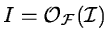is obtained by division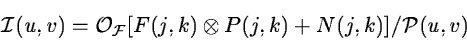(2.27)

if the transformed PSF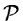is non-zero and the noise N is insignificant. For data with low or medium signal-to-noise ratio (i.e. S/N < 100), as for most optical observations, this technique introduces artifacts in the deconvolved image. These effects can be reduced by filtering the transforms with Wiener filters (Helstrom 1967, Horner 1970).

Another group of image restoration algorithms use iterative methods to obtain a solution which is consistent with the data. The maximum entropy method uses either the entropy :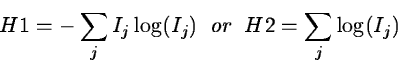(2.28)

in the optimizing procedure (Frieden 1972). It tends to enhance sharp features but a solution may depend on the initial guess and therefore not be unique.

A different scheme was introduced by Lucy (1974) who uses a correction term based on the ratio between the image and the guess. A first guess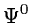must be specified (e.g. a constant) to start the iteration. The first step in the iteration performs a convolution with the PSF :(2.29)

The second step computes a correction factor based on this frame and the original image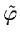: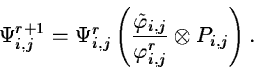(2.30)

The procedure repeats these two steps until the corrections are sufficiently small. After Equation 2.30 is computed the iteration continues with Equation 2.29. This scheme reaches a stable solution very quickly (i.e. 3-5 steps) and is little affected by noise. This makes it very useful for low signal-to-noise data. A photographic picture of a galaxy is used to illustrate this technique (see Figure 2.11). A fit to the stellar image was used to define the PSF.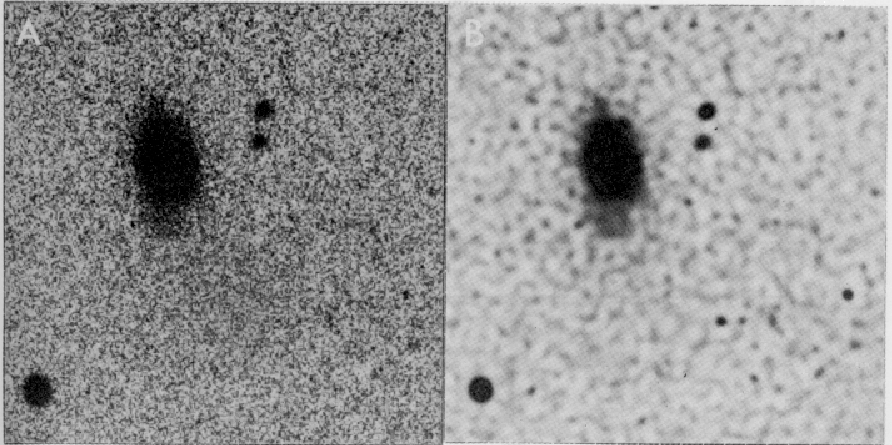Next: Extraction of Information Up: Image Manipulations Previous: Transformations
Petra Nass
1999-06-15